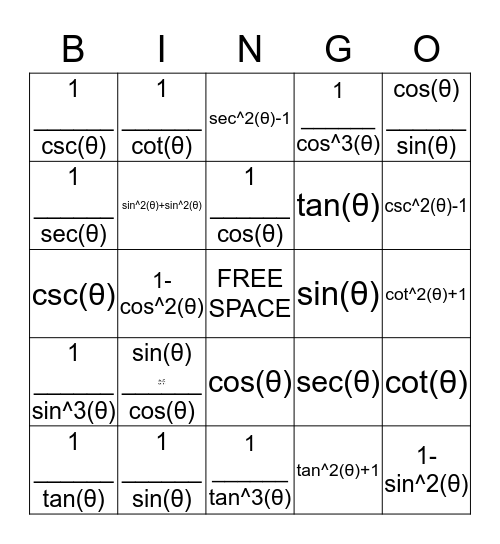# Trigonometry Identities Bingo!This bingo card has 2 images, a free space and 24 words: sin⁡(θ) ______ cos(θ), 1 ______ sin(θ), 1 ______ cos(θ), 1 ______ tan(θ), cos(θ) ______ sin(θ), 1 ______ csc(θ), 1 ______ sec(θ), 1 ______ cot(θ), sin(θ), cos(θ), tan(θ), csc(θ), sec(θ), cot(θ), sin⁡^2(θ)+sin^2⁡(θ), tan^2(θ)+1, cot^2(θ)+1, sec^2(θ)-1, csc^2(θ)-1, 1-cos^2(θ), 1-sin^2(θ), 1 ______ sin^3(θ), 1 ______ cos^3(θ) and 1 ______ tan^3(θ).

## Play Online